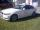Ski class

Class attend 30 boys and some number of girls. Ski training was attended by 28 boys and all the girls, which was 95% of all students. How many girls attend this class? How many percent is that?

Result

x =  10
p =  25 %

Solution:

28 + x = 95/100*(30+x)

5x = 50

x = 10

Calculated by our simple equation calculator.Leave us a comment of this math problem and its solution (i.e. if it is still somewhat unclear...):Be the first to comment!To solve this verbal math problem are needed these knowledge from mathematics:

Our percentage calculator will help you quickly calculate various typical tasks with percentages. Do you have a linear equation or system of equations and looking for its solution? Or do you have quadratic equation?

Next similar math problems:

1. ClassIn a class are 32 pupils. Of these are 8 boys. What percentage of girls are in the class?
2. PupilsThere are 350 girls in the school, and the other 30% of the total number of pupils are boys. How many pupils does the school have?
3. GirlsThe children's competition was attended by 63 girls, which is 30% of all children's participants. How many children attended this competition?
4. Driving schoolDriving final exam involving 10 attendants. The exam consists of three parts. 2 fails at test (quiz) and 6 students fails on car skills test on playground (slalom between the cone). The practical part of the test - driving in town in the real traffic - al
5. Unknown numberIdentify unknown number which 1/5 is 40 greater than one tenth of that number.
6. Reducing numberReducing the an unknown number by 28.5% we get number 243.1. Determine unknown number.
7. Percentage - fractionsAbout what percentage we must increase number 1/6 to get number 1/3?
8. Base, percents, valueBase is 344084 which is 100 %. How many percent is 384177?
9. Seeds 2How many seeds germinated from 1000 pcs, when 23% no emergence?
10. Percents - easyHow many percent is 432 out of 434?
11. GlovesI have a box with two hundred pieces of gloves in total, split into ten parcels of twenty pieces, and I sell three parcels. What percent of the total amount I sold?
12. FactoryIn the factory workers work in three shifts. In the first inning operates half of all employees in the second inning and a third in the third inning 200 employees. How many employees work at the factory?
13. ShotsDetermine the percentage rate of keeper interventions if from 32 shots doesn't caught four shots.
14. PersonsPersons surveyed:100 with result: Volleyball=15% Baseball=9% Sepak Takraw=8% Pingpong=8% Basketball=60% Find the average how many like Basketball and Volleyball. Please show your solution.
15. EnrollmentThe enrollment at a local college increased 4% over last year's enrollment of 8548. Find the increase in enrollment (x1) and the current enrollment (x2).
16. Sales offGoods is worth € 70 and the price of goods fell two weeks in a row by 10%. How many % decreased overall?
17. CandyPeter had a sachet of candy. He wanted to share with his friends. If he gave them 30 candies, he would have 62 candies. If he gave them 40 candies, he would miss 8 candies. How many friends did Peter have?